##### Chemistry For Dummies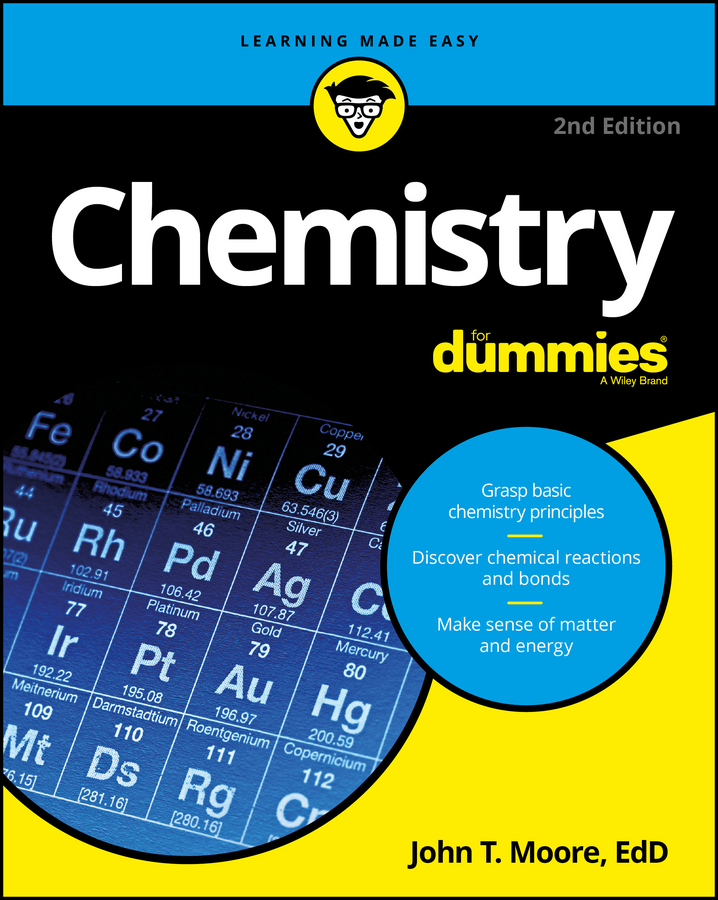Chemistry may not be much of a race, but what if you need to draw a Lewis structure as quickly as you can? Here’s a step-by step process to make it happen, along with an example showing how you would apply these steps to draw the structure of water:

1. Write a skeletal structure showing a reasonable bonding pattern using just the element symbols.

In the case of water, H2O, oxygen is the central element and the hydrogen atoms are both bonded to it. The bonding pattern looks like this: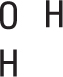2. Take all the valence electrons from all the atoms and throw them into an electron pot.

Each hydrogen atom has 1 electron, and the oxygen atom has 6 valence electrons (VIA family), so you have 8 electrons in your electron pot.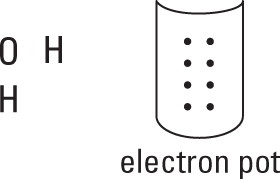3. Use the N – A = S equation to compute the number of bonds in this molecule.

In this equation,

• N equals the sum of the number of valence electrons needed by each atom.

• A is the number of valence electrons in your electron pot — the sum of the number of valence electrons available for each atom.

• S equals the number of electrons shared in the molecule; each bond involves two electrons.

So in the case of water,

• N = 8 + 2(2) = 12 (8 valence electrons for the oxygen atom, plus 2 each for the two hydrogen atoms)

• A = 6 + 2(1) = 8 (6 valence electrons for the oxygen atom, plus 1 for each of the two hydrogen atoms)

• S = 12 – 8 = 4 (four electrons shared in water), and S/2 = 4/2 = 2 bonds

4. Distribute the electrons from your electron pot to account for the bonds.

You use 4 electrons from the 8 in the pot, which leaves you with 4 to distribute later. At least one bond must connect your central atom to the atoms surrounding it.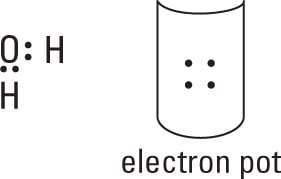5. Distribute the rest of the electrons (normally in pairs) so each atom achieves its full octet of electrons.

Here is the completed electron-dot formula for wate.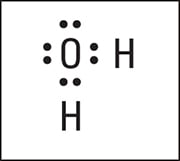Electron-dot formula of H2O.